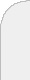inicioir al DIIbuscara probabilistic lexicographic model A lexicographic rule orders alternatives over attributes by first evaluating on the most important attribute and then, if there are ties, on the second most important attribute, and so forth. Previous research has specified mathematical representations and inference procedures of lexicographic rules. However, these methods assume that subjects make deterministic decisions when using a lexicographic rule.  We extend the previous literature by exploring the possibility of probabilistic decisions by subjects using a lexicographic rule.We present a model where subjects are allowed to probabilistically choose an alternative in a choice set when using the lexicographic rule. The model permits assessing the degree of use of the lexicographic rule, and obtains a probabilistic ordering of the attributes at the individual level. We overcome the usual lack of sufficient information from choice data by pooling the data across subjects using a hierarchical Bayesian methodology.We validate our model by first simulating data and analyzing model identification and parameter recovery and then implementing the model in a conjoint-based dataset. We propose a MCMC methodology to estimate the model's parameters. We compare fit and prediction ability of the proposed method with competing models. Results show that a substantial fraction of the subjects in our study use a lexicographic rule.